PPWIZARD is a free preprocessor for HTML, REXX, Visual Basic or any text files.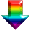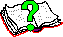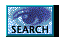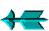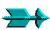\ -> Rexx -> Inbuilt PPWIZARD Functions -> ArrayReverse()

 ArrayReverse()

This is an inbuilt function function provided by PPWIZARD.

This function is used to reverse the order of elements in an array. The "array" has numeric indexes with the ".0" element holding the number of elements.

The function takes a single parameter which is the name of the array (without dot).

The routine returns the number of items in the array.

 Silly Example

```;--- Set up the array -----------------------
#evaluate ''               \
^                \
A.1 = "5345";  \
A.2 = "4123";  \
A.3 = "61743"; \
A.4 = "1678";  \
A.0 = 4;       \
^

;--- Sort array & Reverse order & display ---
#evaluate '' ^call ArraySort "A"^
#evaluate '' ^call ArrayReverse  "A"^
#evaluate '' ^do I = 1 to A.0; say a.i; end;^
```

Saturday May 28 2022 at 2:55pm